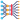# Understanding dimensions in PyTorch

//Edit✏️

When I started doing some basic operations with PyTorch tensors like summation, it looked easy and pretty straightforward for one-dimensional tensors:

``````>> x = torch.tensor([1, 2, 3])
>> torch.sum(x)
tensor(6)``````

However, once I started to play around with 2D and 3D tensors and to sum over rows and columns, I got confused mostly about the second parameter `dim` of `torch.sum`.

Let’s start by what the official documentation says:

torch.sum(input, dim, keepdim=False, dtype=None) → Tensor

Returns the sum of each row of the input tensor in the given dimension dim.

I don’t quite understand this explication. We can sum up over columns so why do one mention that it just “returns the sum of each row” ? This was my first incomprehension.

However, the more important problem was, as I said, the direction of each dimension. Here’s what I mean. When we describe the shape of a 2D tensor, we say that it contains some rows and some columns. So for a 2x3 tensor we’ve 2 rows and 3 columns:

``````>> x = torch.tensor([
[1, 2, 3],
[4, 5, 6]
])
>> x.shape
torch.Size([2, 3])``````

We specify at first the rows (2 rows) and then the columns (3 columns), right? That brought me to the conclusion that the first dimension (dim=0) stays for rows and the second one (dim=1) for columns. Following the reasoning that the dimension dim=0 means row-wise, I expected `torch.sum(x, dim=0)` to result in a `1x2` tensor (`1 + 2 + 3` and `4 + 5 + 6` for an outcome of tensor[6, 15]). But it turned out I got something different: a `1x3` tensor.

``````>> torch.sum(x, dim=0)
tensor([5, 7, 9])``````

I was surprised to see that the reality was the opposite of what I’ve expected because I finally got the result tensor[6, 15] but when passing the parameter dim=1:

``````>> torch.sum(x, dim=1)
tensor([6, 15])``````

So why is that? I found out an article of Aerin Kim 🙏 tackling down the same confusion but for NumPy matrices where we pass a second parameter called axis. NumPy sum is almost identical to what we have in PyTorch except that dim in PyTorch is called axis in NumPy:

numpy.sum(a, axis=None, dtype=None, out=None, keepdims=False)

The key to grasp how dim in PyTorch and axis in NumPy work was this paragraph from Aerin’s article:

The way to understand the “axis” of numpy sum is that it collapses the specified axis. So when it collapses the axis 0 (the row), it becomes just one row (it sums column-wise).

She explains very well the functioning of the axis parameter on numpy.sum. However, it becomes trickier when we introduce a third dimension. When we look at the shape of a 3D tensor we’ll notice that the new dimension gets prepended and takes the first position i.e. the third dimension becomes `dim=0`.

``````>> y = torch.tensor([
[
[1, 2, 3],
[4, 5, 6]
],
[
[1, 2, 3],
[4, 5, 6]
],
[
[1, 2, 3],
[4, 5, 6]
]
])

>> y.shape
torch.Size([3, 2, 3]) // Notice the first 3``````

Yes, it’s quite confusing. That’s why I think some basic visualizations of the process of summation over different dimensions will greatly contribute to a better understanding.

The first dimension (dim=0) of this 3D tensor is the highest one and contains 3 two-dimensional tensors. So in order to sum over it we have to collapse its 3 elements over one another:

``````>> torch.sum(y, dim=0)
tensor([[ 3,  6,  9],
[12, 15, 18]])``````

Here’s how it works:For the second dimension (dim=1) we have to collapse the rows:

``````>> torch.sum(y, dim=1)
tensor([[5, 7, 9],
[5, 7, 9],
[5, 7, 9]])``````And finally, the third dimension (dim=2) collapses over the columns:

``````>> torch.sum(y, dim=2)
tensor([[ 6, 15],
[ 6, 15],
[ 6, 15]])``````If you’re like me, recently started to learn PyTorch or NumPy, I hope these basic animated examples will help you to get a better understanding of how dimensions work, not only for sum but for other methods as well.